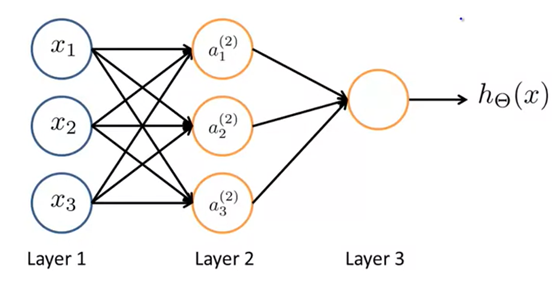# The Workflow of Neural Network Explained

Original article was published on Artificial Intelligence on Medium# The Workflow of Neural Network Explained

## This article explains the workflow and formulas of neural networks using the backpropagation method.

Neural Network has been developed to mimic a human brain. Though we are not there yet, neural networks are very efficient in machine learning. It was very popular in the 1980s and 1990s. Recently it has become more popular. Computers are fast enough to run a large neural network in a reasonable time. In this article, I will discuss how a neural network works.

## Ideas of Neural Network

In a simple neural network, neurons are the basic computation units. They take the input features and channel them out as output. Here is how a basic neural network looks like: